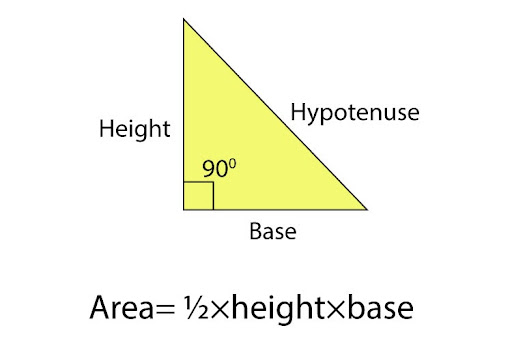# How do I work out the area of a right-angled triangle?

In a right angle triangle, each leg represents a different measurement. The vertical distance from the base of the 90 degree angle to the tip of the triangle is referred to as the height, while the horizontal distance is referred to as the base. The slant length from the tip of the height to the base is identified as the hypotenuse. However, when calculating the area of a right angle triangle, we will only be focusing on the base and the height of the triangle in question.

E.g. Case 1

In a question they had stated that the height of the triangle was 6 cm and the base was 12 cm and you were asked to find the area of this right angle triangle.
Formula:

Area= ½×height×base

Now you substitute the given values.
Area=½×6×12
=36 cm²
Case 2
There are instances where they don’t give you the value of the height or base, but instead identify the value of the hypotenuse and one other length. In this case, you will need to apply the Pythagorean Theorem.
Formula:
(hypotenuse)² = (height)² + (base)²
Let’s say in a question they have given you the value of the hypotenuse is 13 cm and that of the base is 5 cm. The question is asking you to calculate the area of this right-angle triangle.
We begin with finding the height because without that you cannot apply the area formula. In order to find the height, we need to apply the Pythagorean theorem. You begin identifying the present and the missing values. Then we substitute each present value and find the missing one, which in this case is the height.
(13)² = (height)² + (5)²
169 = (height)² + 25
169 - 25= (height)²
144= (height)² ⇒ Take square root on both sides in this step
12 cm = height
Now we use the value of height and base to find the area of this right angle triangle.
Area = ½×12×5
= 30 cm²